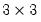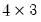graph
Create named graph object containing the results of a graph command, or created when merging multiple graphs into a single graph.
Syntax
graph graph_name.graph_command(options) arg1 [arg2 arg3 ...]
graph graph_name.merge graph1 graph2 [graph3 ...]
Follow the keyword with a name for the graph, a period, and then a statement used to create a graph. There are two distinct forms of the command.
In the first form of the command, you create a graph using one of the graph commands, and then name the object using the specified name. The portion of the command given by,
graph_command(options) arg1 [arg2 arg3 ...]
should follow the form of one of the standard EViews graph commands:

 area Area graph ( area). band Area band graph ( band). bar Bar graph ( bar). boxplot Boxplot graph ( boxplot). distplot Distribution graph ( distplot). dot Dot plot graph ( dot). errbar Error bar graph ( errbar). hilo High-low(-open-close) graph ( hilo). line Line graph ( line). pie Pie graph ( pie). qqplot Quantile-Quantile graph ( qqplot). scat Scatterplot—same as XY, but lines are initially turned off, symbols turned on, and aframe is used ( scat). scatmat Matrix of scatterplots ( scatmat). scatpair Scatterplot pairs graph ( scatpair). seasplot Seasonal line graph ( seasplot). spike Spike graph ( spike). xyarea XY line-symbol graph with one X plotted against one or more Y’s using existing line-symbol settings ( xyarea). xybar XY line-symbol graph with one X plotted against one or more Y’s using existing line-symbol settings ( xybar). xyline Same as XY, but symbols are initially turned off, lines turned on, and aframe is used ( xyline). xypair Same as XY but sets XY settings to display pairs of X and Y plotted against each other ( xypair).
In the second form of the command, you instruct EViews to merge the listed graphs into a single graph, and then name the graph object using the specified name.
Options

 reset Resets all graph options to the global defaults. May be used to remove existing customization of the graph. p Print the graph (for use when specified with a graph command).
Additional options will depend on the type of graph chosen. See the entry for each graph type for a list of the available options (for example, see bar for details on bar graphs).
Examples
graph gra1.line(s, p) gdp m1 inf
creates and prints a stacked line graph object named GRA1. This command is equivalent to running the command:
line(s, p) gdp m1 inf
freezing the view, and naming the graph GRA1.
graph mygra.merge gr_line gr_scat gr_pie
creates a multiple graph object named MYGRA that merges three graph objects named GR_LINE, GR_SCAT, and GR_PIE.
Cross-references
See “Graph Objects” for a general discussion of graphs.